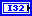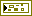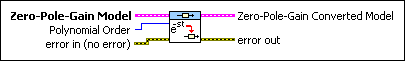# CD Convert Delay with Pade Approximation VI

LabVIEW 2018 Control Design and Simulation Module Help

Edition Date: March 2018

Part Number: 371894J-01

»View Product InfoDownload Help (Windows Only)

Owning Palette: Model Conversion VIs

Requires: Control Design and Simulation Module

Incorporates time delays in a continuous-time system model using Pade approximation, which converts all residuals. Wire data to the State-Space Model input to determine the polymorphic instance to use or manually select the instance.

Use the pull-down menu to select an instance of this VI.

 Select an instance CD Convert Delay with Pade Approximation (State-Space)CD Convert Delay with Pade Approximation (Transfer Function)CD Convert Delay with Pade Approximation (Zero-Pole-Gain)

## CD Convert Delay with Pade Approximation (State-Space)State-Space Model contains a mathematical representation of and information about the continuous-time system for which this VI incorporates time delays using Pade approximation.Polynomial Order is the order of the Pade approximation polynomials. The default value is 3.error in describes error conditions that occur before this node runs. This input provides standard error in functionality.State-Space Converted Model returns the system for which this VI incorporates the total time delay between each input-output pair into the transfer function between that pair using Pade approximation of Polynomial Order. To access and modify the data in the model, use the Model Information VIs.error out contains error information. This output provides standard error out functionality.

## CD Convert Delay with Pade Approximation (Transfer Function)Transfer Function Model contains a mathematical representation of and information about the continuous-time system for which this VI incorporates time delays using Pade approximation.Polynomial Order is the order of the Pade approximation polynomials. The default value is 3.error in describes error conditions that occur before this node runs. This input provides standard error in functionality.Transfer Function Converted Model is the system for which this VI incorporates the total time delay between each input-output pair into the transfer function between that pair using Pade approximation of Polynomial Order. To access and modify the data in the model, use the Model Information VIs.error out contains error information. This output provides standard error out functionality.

## CD Convert Delay with Pade Approximation (Zero-Pole-Gain)Zero-Pole-Gain Model contains a mathematical representation of and information about the continuous-time system for which this VI incorporates time delays using Pade approximation.Polynomial Order is the order of the Pade approximation polynomials. The default value is 3.error in describes error conditions that occur before this node runs. This input provides standard error in functionality.Zero-Pole-Gain Converted Model is the system for which this VI incorporates the total time delay between each input-output pair into the transfer function between that pair using Pade approximation of Polynomial Order. To access and modify the data in the model, use the Model Information VIs.error out contains error information. This output provides standard error out functionality.